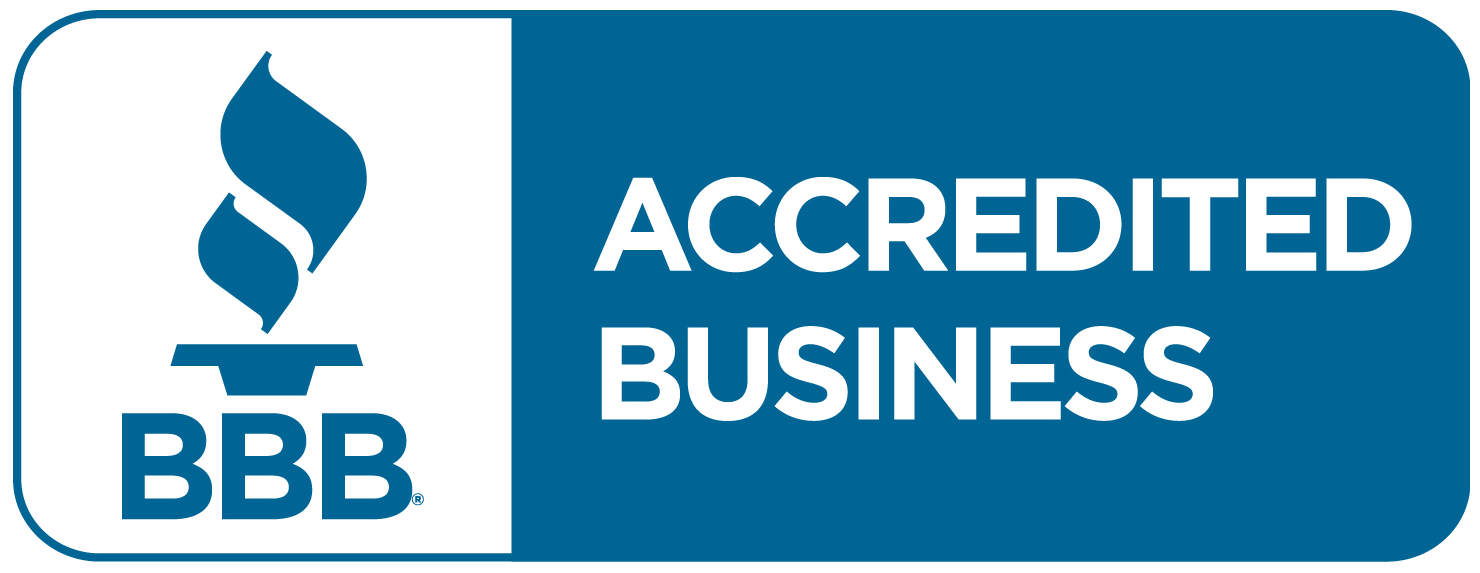Straight Line Depreciation

# Straight Line Depreciation Definition

The straight line method of depreciation is the simplest method of depreciation. Using this method, the cost of a tangible asset is expensed by equal amounts each period over its useful life. The idea is that the value of the assets declines at a constant rate over its useful life. This method is approved by GAAP.

## Straight Line Depreciation Formula

To calculate straight line depreciation for an asset, you need the asset’s purchase price, salvage value, and useful life. The salvage value is the amount the asset is worth at the end of its useful life. Whereas the depreciable base is the purchase price minus the salvage value. Depreciation continues until the asset value declines to its salvage value.
First, calculate the depreciable base. Then divide the depreciable base by the useful life. You will find the depreciation expense used for each period until the value of the asset declines to its salvage value.

Depreciable Base = Purchase Price – Salvage Value

Depreciation Expense = Depreciable Base / Useful Life

For example, if a company purchased an asset for \$100, then its salvage value is \$0 and its useful life is 10 years. Then the annual depreciation expense for the asset would be \$10 (10 = 100/10). Therefore, after 10 years, the asset will have depreciated to its salvage value – in this example \$0. Thus, the depreciation for the asset will no longer occur.

### Straight Line Depreciation Rate

The depreciation rate is the rate an asset is depreciated each period. To calculate the depreciation rate, divide the depreciation expense by the depreciable base. To find the depreciation expense using the deprecation rate, multiply the depreciable base by the depreciation rate.

Depreciation Rate = Depreciation Expense / Depreciable Base

Depreciation Expense = Depreciation Rate x Depreciable Base

In the above example, the depreciation rate would be 10% (10% = 10/100).ARTICLES YOU MIGHT LIKE

### The Struggles of Private Company Accounting

Hiring the right accountant  When I meet a business owner operating at a successful \$10 million in revenue, they often mention, “My CPA”… I immediately know that CEO/Entrepreneur is referring to their Tax CPA.  That is because one thing that all Entrepreneurs have in common is that they must file a tax return.  So, from

### IN CRISIS? GET A STRATEGIC CFO! – CEO Blindspots Podcast

Friend of the firm, Birgit Kamps, recently had Strategic CFO President, Dan Corredor, as a guest on her podcast, CEO Blindspots. CEO BLINDSPOTS HOST: Birgit Kamps. She was speaking five languages by the age of 10, and lived in five countries with her Dutch parents prior to becoming an American citizen. Birgit’s professional experience includes starting

### SHRM calls ICHRA the 401K for Group Health Benefits

Fed-up with group health insurance? ICHRA is the new way to offer great health benefits and avoid ACA penalties, SHRM calls it the 401K for group health benefits.  In 2020 the Department of Labor, HHS and IRS changed the rules for employer health benefits. They changed the Affordable Care Act mandates and penalties for every

MARCH 28TH-31ST 2022

JOIN THE NEXT STRATEGIC CFO™ SERIES

The Art of the CFO®

Days
Hours
Min

September 12-15th 2022